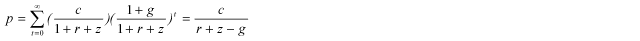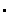Appended Note 1-6 Estimation method of profit-return price index

1. Concept of profit-return method
The profit-return method is a method in which the asset price of the subject real estate is determined by summing up the current value of the net income the subject real estate is expected to generate in the future. The profit-return price is calculated from the relational expression:
Profit-return price = (net income for a given period)/(capitalization yield)
This is the so-called direct return method of the profit-return method where the current value is derived by rebating a fixed rate of the income gained from the subject real estate for a given period. In particular, the profit-return price p is calculated with the following equation under the hypothesis that the future net income will increase with the fixed rate g (expected growth rate), and while having the net income of the current term represented by c, the interest rate of safe assets by r and the risk premium by z.The above equation indicates that the profit-return price is monotonically increasing relative to the expected growth rate (g), while it is monotonically decreasing relative to the interest rate of safe assets (r) and the risk premium (z). In other words, the profit-return price increases when capitalization yield decreases.

2. Estimation method of profit-return price index of commercial real estates in commercial zones in the center of each city
In the "Japanese Real Estate Investor Survey" of the Japan Real Estate Institute (JREI), a questionnaire survey on the expected yields and other factors was conducted with the assumption that the real estate in the region determined to be the commercial zones in the center of each city will be purchased as real estate for investment. Based on the results of this survey, a simplified model based on the profit-return model was used to examine the trend of the profit-return price amidst the changing rent and expected yields while with the following premises.Interest rate of safe assets (r) and risk premium (z): The "expected cap rate" of each city in the "Japanese Real Estate Investor Survey" was used as the total of the above two factors (r) and (z).While net income is the amount of rent revenue from which maintenance fee and other expenses have been subtracted, the total rent income has been used as a substitute since data on expenses were difficult to collect. The total rent revenue has been established based on the rent index of the region closest to the region determined in the "Japanese Real Estate Investor Survey" out of all the choices given in the "Office rent index in the major regions of Japan" section of the "Office and Apartment Rent Index" of the JREI.Expected growth rate of new income (g): Established based on the "Office rent forecast" for each city in the "Japanese Real Estate Investor Survey."
The profit-return price index between 2002 and 2004 was estimated with the above assumptions and while determining the profit-return price of 2001 as 100. Furthermore, the values are based on that at the end of September of each year.